# Extensible T-Deck Lisp Editor

#1

This article describes an updated version of the T-Deck Lisp Editor. This second version is functionally identical to the first version, but it has been improved to make it easier to extend it by adding your own editing commands.

For example, you can now add a command g to insert a ‘>’ symbol in your program simply by evaluating:

``````(define-atomic-cmd #\g "greater" #'(lambda (fun) (pop *cmds*) (%edit (cons '> fun))))
``````

These updates make the T-Deck Lisp Editor a bit like a baby Emacs.

Here’s the source of the Extensible T-Deck Lisp Editor: Extensible Lisp Editor.

It encodes the string as a C++ Raw String Literal to avoid needing to enclose each line in double quotes, and to escape special characters within the strings. Thanks to @nanomonkey for suggesting this improvement.

For information about installing it and using it see the earlier article: A Lisp Editor for T-Deck.

### How the editor works

The main edit function reads the key presses you type, and rejects any illegal key presses. It builds up a list of the editing commands you have given in the global variable *cmds*.

After each key press it calls %edit, which executes the list of editing commands on the function (or variable) you are editing, and prettyprints the result, using pprint.

Here’s the function %edit, that executes the list of editing commands in *cmds*:

``````(defun %edit (fun)
(let ((cmd (car *cmds*)))
(cond
((null *cmds*) fun)
((eq cmd #\h) (pop *cmds*) (%edit (cons 'highlight (list fun))))
((consp cmd) (funcall (cdr (assoc (car cmd) *binary-cmds*)) (cdr cmd) fun))
((assoc cmd *atomic-cmds*) (funcall (cdr (assoc cmd *atomic-cmds*)) fun))
(t fun))))
``````

There are two types of editing commands:

• Atomic (single-key) commands. These are represented by a single character, such as #\d.
• Binary commands that take a parameter. These are represented by a cons, consisting of the editing key followed by the argument, such as (#\r . +).

The single-key commands are defined in the association list *atomic-cmds*, and the binary commands are defined in the association list *binary-cmds*.

You can see the effect of %edit by setting *cmds* to a list of editing commands, and then calling it on a list. For example, suppose we have the list:

``````(lambda (x) (* x x))
``````

The following edit sequence changes the ‘*’ to a ‘+’:

``````(setq *cmds* '(#\d #\d #\a #\a (#\r . +)))
``````

Trying it out:

``````> (setq *cmds* '(#\d #\d #\a #\a (#\r . +)))
(#\d #\d #\a #\a (#\r . +))

> (%edit '(lambda (x) (* x x)))
(lambda (x) (+ x x))
``````

#### Processing the editing commands

The function %edit is called for each of the editing commands in the *cmds* list. It first sets the local variable cmd to the first command in the list, and a cond structure then selects the appropriate action for that command.

For example, the action for the x (delete) command is defined by the following entry in the *atomic-cmds* association list:

``````(#\x . #'(lambda (fun) (pop *cmds*) (if (atom fun) (%edit fun) (%edit (cdr fun)))))
``````

This first pops the completed command off the front of the *cmds* list.

It then checks whether the current selection fun is an atom, in which case the command is invalid and simply calls %edit on fun again.

Otherwise it calls %edit on the cdr of fun, effectively deleting the car.

#### The main edit function

The main function edit prettyprints the function being edited, and then waits for a key press.

It handles the special editing commands, such as q (quit), s (save) and z (undo), that don’t get passed to %edit.

If the key is in the *binary-cmds* or *atomic-cmds* association lists it adds it to the command buffer.

It then calls %edit to apply the editing commands in *cmds* to the function, and loops around to print the result.

### Adding commands to the editor

The functions define-atomic-cmd and define-binary-cmd are provided as a convenient way of adding commands to the corresponding association lists of commands:

``````(defun define-atomic-cmd (char name cmd) (push (cons char cmd) *atomic-cmds*))
(defun define-binary-cmd (char name cmd) (push (cons char cmd) *binary-cmds*))
``````

For example, to define the x (delete) command already described, you call:

``````(define-atomic-cmd #\x "delete"
#'(lambda (fun) (pop *cmds*) (if (atom fun) (%edit fun) (%edit (cdr fun)))))
``````

You can use these commands to define a new editing command and add it to the Lisp Editor.

#### Defining your own editing command

As an example, let’s define an editing command w that swaps the next two forms after the editor cursor (we can’t call it s because that is already used for save).

For example, it will be useful if we’ve tried to define a function big that tests whether its argument is greater than 100, but we’ve written it incorrectly as:

``````(defun big (a) (> 100 a))
``````

In the Lisp Editor we will be able to move the green block cursor in front of 100 and press w.

To define the swap command execute the following:

``````(define-atomic-cmd #\w "swap"
#'(lambda (fun)
(pop *cmds*)
(cond
((null (cdr fun)) (%edit fun))
(t (%edit (cons (second fun) (cons (first fun) (cddr fun))))))))
``````

If there aren’t two forms after the cursor (cdr fun) is nil, and we ignore the command.

Otherwise we call %edit on the current value of fun with the first two items swapped.

Let’s try it out:

• Type d d a d to position the block cursor: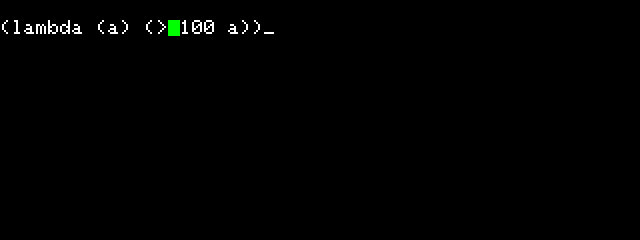• Type w to swap the next two items: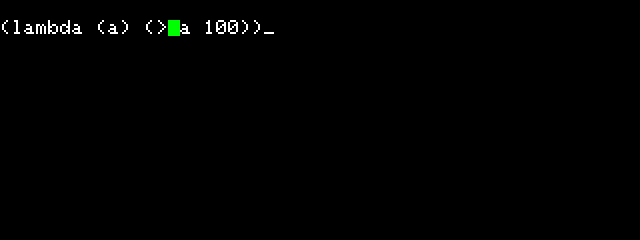• Type s to save the corrected version.

#### A second example

Here’s a second example. It defines a command p, for prototype, that inserts a function call prototype into your program at the block cursor position. It’s useful if you can’t remember the correct arguments for a function, or what order they should be in.

For example, if you want to insert a call to subseq into the program you are editing, position the cursor at the correct position and type p.

The Lisp Editor prompts for the name of the function you want to insert:

Here’s the definition:

``````(define-binary-cmd #\p "prototype"
#'(lambda (val fun)
(pop *cmds*)
(%edit (cons (read-from-string (documentation val)) fun))))
``````

For example, we have defined an initial attempt at a function to print the day name:

``````(defun day (n)
(let ((days "MonTueWedThuFriSatSun"))
(print)))
``````

We want to insert the correct function call after print.

• Type:

``````(edit 'day)
``````
• Type d d a d d a d to position the block cursor after print.

• Type p followed by subseq: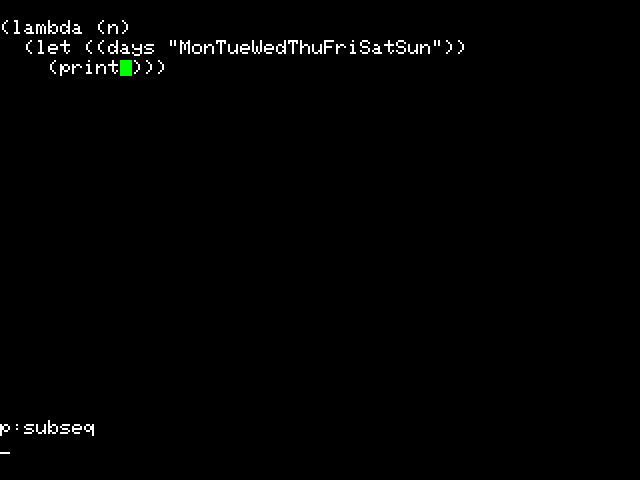The p command inserts the prototype for subseq at the cursor position: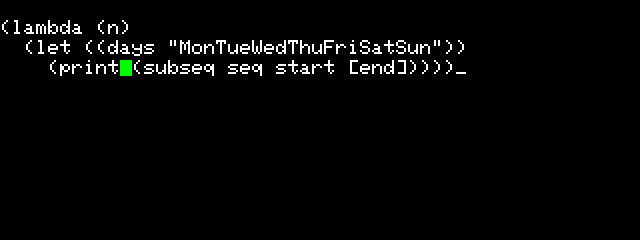• Type a d a to select seq and type r days to replace it with days: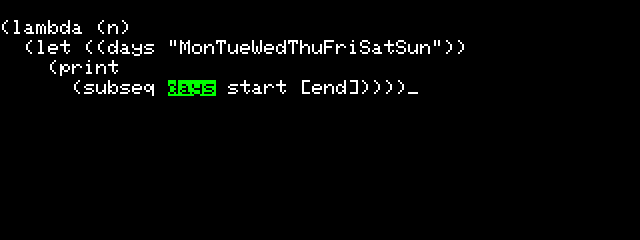• Type b d a to select start and type r (* n 3) to replace it with (* n 3):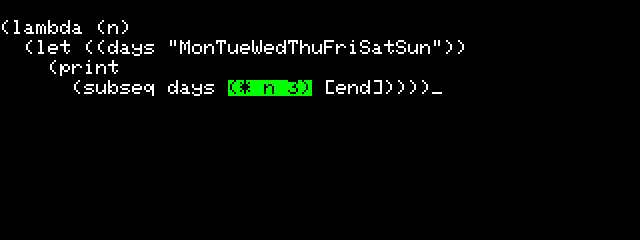• Type b d a to select [end] and type r (* (1+ n) 3) to replace it with (* (1+ n) 3):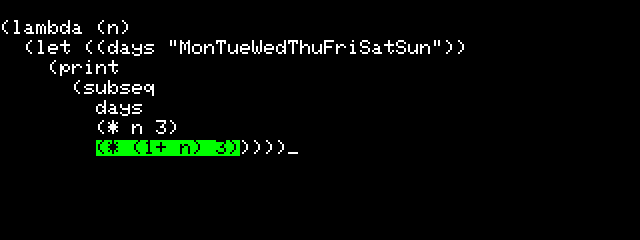• Finally type s to save the edited function.

Checking it works correctly:

``````> (day 4)
"Fri"
``````

A Lisp Editor for T-Deck
#2

I added scrolling again but this time I got it to work much smoother and faster for long functions. The commands are the same as last time

• u scroll up by 1 line
• j scroll down by 1 line
• y scroll up by 5 lines
• h scroll down by 5 lines

The trick is that I modified the search function to be able to search for the nth repeat of a pattern. This function is in C so you have to use the extensions.ino method to add it, while the rest of the editor uses the lisp library method

``````object *fn_searchn (object *args, object *env) {
(void) env;
int matches = 1;
int last_index = 0;
object *pattern = first(args);
object *target = second(args);
if (cddr(args) != NULL){
object *num = third(args);
if(integerp(num)){
matches = num->integer;
}
}
if (pattern == NULL) return number(0);
else if (target == NULL) return nil;
else if (listp(pattern) && listp(target)) {
int l = listlength(target);
int m = listlength(pattern);
for (int i = 0; i <= l-m; i++) {
object *target1 = target;
while (pattern != NULL && eq(car(target1), car(pattern))) {
pattern = cdr(pattern);
target1 = cdr(target1);
}
if (pattern == NULL){
last_index = i;
if(--matches <= 0){
return number(i);
}
}
pattern = first(args); target = cdr(target);
}
if(last_index > 0){
return number(last_index);
}
return nil;
} else if (stringp(pattern) && stringp(target)) {
int l = stringlength(target);
int m = stringlength(pattern);
for (int i = 0; i <= l-m; i++) {
int j = 0;
while (j < m && nthchar(target, i+j) == nthchar(pattern, j)) j++;
if (j == m){
last_index = i;
if(--matches <= 0){
return number(i);
}
}
}
if(last_index > 0){
return number(last_index);
}
return nil;
} else error2(PSTR("arguments are not both lists or strings"));
return nil;
}

const char docsearchn[] PROGMEM = "(searchn pattern target [n])\n"
"Returns the index of the nth occurrence of pattern in target,\n"
"which can be lists or strings, or nil if it's not found.\n"
"if the pattern occured more than once but less than n times, it returns the last occuring index";

//{ stringsearchn, fn_searchn, 0223, docsearchn }
``````

So now our line trimming functions are really straightforward because its easy to find the nth occurrence of the newline character

``````(defun linecut (str n)
"cuts off the first n lines of a string"
(subseq str (1+ (searchn
(string (code-char 10)) str n))))

(defun linekeep (str n)
"keeps the first n lines of a string"
(subseq str 0 (searchn (string (code-char 10)) str n)))

(defun trim-by-lines (str starting-line span)
"trims the string to a certain amount of lines"
(linekeep (linecut str starting-line) span))
``````

And here’s the modified version of the editor code with the scroll commands (I also brought back undo, also the syntax highlighting gets messed up)

``````(defun %edit (fun)
(let ((cmd (car *cmds*)))
(cond
((null *cmds*) fun)
((eq cmd #\h) (pop *cmds*) (%edit (cons 'highlight (list fun))))
((consp cmd) (funcall (cdr (assoc (car cmd) *binary-cmds*)) (cdr cmd) fun))
((assoc cmd *atomic-cmds*) (funcall (cdr (assoc cmd *atomic-cmds*)) fun))
(t fun))))

(defun butlast (lst) (subseq lst 0 (1- (length lst))))

(defun edit (name)
(let ((fun (eval name))
cc
processed
trimmed
(scroll-pos 0))
(setq *cmds* nil)
(loop
(setq cc (append cc (list #\h)))
(setq *cmds* cc)
(setq processed (with-output-to-string (str) (pprint (%edit fun) str)))
(setq trimmed (trim-by-lines processed scroll-pos 20))
(write-byte 12)
(princ (concatenate 'string trimmed (string #\ETX)))
(setq cc (butlast cc))
(let ((c (get-key)))
(cond
((eq c #\q) (return name))
((eq c #\s) (setq *cmds* cc) (set name (%edit fun)) (return name))
((eq c #\z) (when cc (setq cc (butlast cc))))
((eq c #\u)  (when (< 0 scroll-pos) (setq scroll-pos (1- scroll-pos ))))
((eq c #\j)  (setq scroll-pos (1+ scroll-pos )))
((eq c #\y)  (if (< 5 scroll-pos) (setq scroll-pos (- scroll-pos 5 ))
(setq scroll-pos 0)))
((eq c #\h)  (setq scroll-pos (+ scroll-pos 5)))
((assoc c *binary-cmds*)
(write-byte 11) (princ c) (princ #\:)
(setq cc (append cc (list (cons c (read))))))
((assoc c *atomic-cmds*) (setq cc (append cc (list c))))
(t (write-byte 7)))))))

(defun define-atomic-cmd (char name cmd) (push (cons char cmd) *atomic-cmds*))

(defun define-binary-cmd (char name cmd) (push (cons char cmd) *binary-cmds*))

(define-atomic-cmd #\b "back"
#'(lambda (fun) (pop *cmds*) fun))

(define-atomic-cmd #\d "cdr"
#'(lambda (fun) (pop *cmds*) (if (atom fun) (%edit fun) (%edit (cons (car fun) (%edit (cdr fun)))))))

(define-atomic-cmd #\a "car"
#'(lambda (fun) (pop *cmds*) (if (atom fun) (%edit fun) (%edit (cons (%edit (car fun)) (cdr fun))))))

(define-atomic-cmd #\x "delete"
#'(lambda (fun) (pop *cmds*) (if (atom fun) (%edit fun) (%edit (cdr fun)))))

(define-binary-cmd #\r "replace"
#'(lambda (val fun) (pop *cmds*) (if (atom fun) (%edit val) (%edit fun))))

(define-binary-cmd #\c "cons"
#'(lambda (val fun) (pop *cmds*) (%edit (cons val fun))))

(define-binary-cmd #\i "insert"
#'(lambda (val fun) (pop *cmds*) (%edit (cons val fun))))

(define-binary-cmd #\f "find"
#'(lambda (val fun)
(cond
((null fun) nil)
((equal val fun) (pop *cmds*) (%edit fun))
((atom fun) fun)
(t (cons (%edit (car fun)) (%edit (cdr fun)))))))
``````

#3

Thanks for sharing that! Note that to avoid inappropriate syntax colouring in sections of Lisp code use:

````text
(lisp code)
````